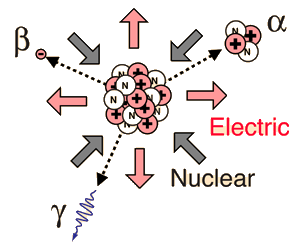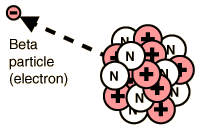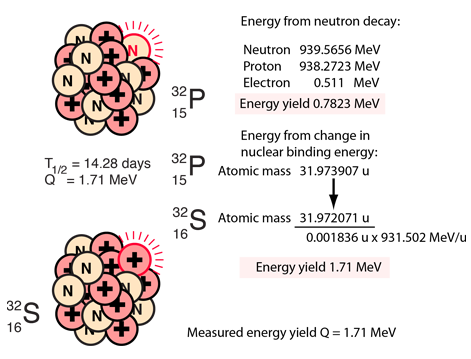Index

 HyperPhysics***** Nuclear R Nave
Go BackBeta particles are just electrons from the nucleus, the term "beta particle" being an historical term used in the early description of radioactivity. The high energy electrons have greater range of penetration than alpha particles, but still much less than gamma rays. The radiation hazard from betas is greatest if they are ingested. Beta emission is accompanied by the emission of an electron antineutrino which shares the momentum and energy of the decay.
 The emission of the electron's antiparticle, the positron, is also called beta decay. Beta decay can be seen as the decay of one of the neutrons to a proton via the weak interaction. The use of a weak interaction Feynman diagram can clarify the process.Electron and antineutrino Positron and neutrino. Energy spectrum.
 Decay of the neutron Fermi theory of beta decay
 How Does the Beta Electron Escape All Those Protons?
Index

Beta decay concepts

 HyperPhysics***** Nuclear R Nave
Go Back

# How Does the Beta Electron Escape All Those Protons?Even though this is not plausible physically (e.g., the electron is not a point particle, it cannot be localized at such a point, etc.) it is an interesting conceptual question. An estimate of the electrical binding energy if it could be placed at the surface of the nucleus may be made by treating the 32P nucleus as a sphere of charge and using the nuclear size model to calculate its radius. Then the electric potential energy could be calculated.

For a mass number A = 32 for 32P , the nuclear size model yields r = 3.8 x 10-15 m.

For 32P with 15 protons, the nuclear charge Q = 2.40 x 10-18 coulombs.

Using the radius and charge, the electric potential energy of the electron would be KQe/r = -9.09 x 10-15 J = -5.68 MeV! It is hard to conceive of an electron escaping that!

Considering that placing an electron at the radius is not plausible, look at the entire beta decay process from the point of view of the nuclear binding energies involved. The process of beta decay must be examined from the point of view of the binding energy difference between the parent and daughter nucleus, and the energy yield is then distributed to the products of the decay.The decay of 32P can be considered to be a collective beta decay process, examined in more detail above. The total measured energy release Q is consistent with the identified nuclear processes. It can be calculated from the difference in neutral atomic masses for 32P and 32S.

The electron from the beta decay of 32P can approach the full decay energy of 1.71 MeV, but it has an average value of about 0.7 MeV.

For another numerical example, consider the example of the beta decay of bismuth 210Bi cited by Krane.

For the beta decay 210Bi -> 210Po

 Qβ- = [m(210Bi) - m(210Po)]c2 =(209.984095 u -209.982848 u)(931.502 MeV/u) = 1.161 MeVFor beta decay, involving three particles, the beta electron energy is distributed from a low value up to the energy yield of the decay. The electron antineutrino carries most of the remaining energy, the recoil energy of the nucleus being very small.
 Electron and antineutrino Positron and neutrino. Energy spectrum.
 Decay of the neutron Fermi theory of beta decay
Index

Beta decay concepts

Krane, Ch 9

 HyperPhysics***** Nuclear R Nave
Go Back

# Electron and Antineutrino

Early studies of beta decay revealed a continuous energy spectrum up to a maximum, unlike the predictable energy of alpha particles. Another anomaly was the fact that the nuclear recoil was not in the the direction opposite the momentum of the electron. The emission of another particle was a probable explanation of this behavior, but searches found no evidence of either mass or charge. The interesting history has Wolfgang Pauli in 1930 proposing an as yet unobserved particle to explain the continuous distribution of energy of the emitted electrons. Then Enrico Fermi called this particle a neutrino and developed a theory of beta decay in which the neutrino carried away the missing energy and momentum. With no charge and almost no mass, it was hard to detect, and not until 1956 was experimental detection of the neutrino achieved. For symmetry reasons, the particle emitted along with the electron from nuclei is called an antineutrino. The emission of a positron is accompanied by a neutrino.

Index

Beta decay concepts

 HyperPhysics***** Nuclear R Nave
Go Back

# Positron and Neutrino

The emission of a positron or an electron is referred to as beta decay. The positron is accompanied by a neutrino, an almost massless and chargeless particle. Positrons are emitted with the same kind of energy spectrum as electrons in negative beta decay because of the emission of the neutrino.Index

Beta decay concepts

 HyperPhysics***** Nuclear R Nave
Go Back

# Beta Energy Spectrum

In the process of beta decay, either an electron or a positron is emitted. Because either a neutrino or an antineutrino is emitted as well, there is a spectrum of energies for the electron or positron, depending upon what fraction of the reaction energy Q is carried by the massive particle. The shape of this energy curve can be predicted from the Fermi theory of beta decay.Experimental energy and momentum distributions
Index

Beta decay concepts

Reference
Halliday, Resnick, Walker
Sec. 47.5

 HyperPhysics***** Nuclear R Nave
Go Back